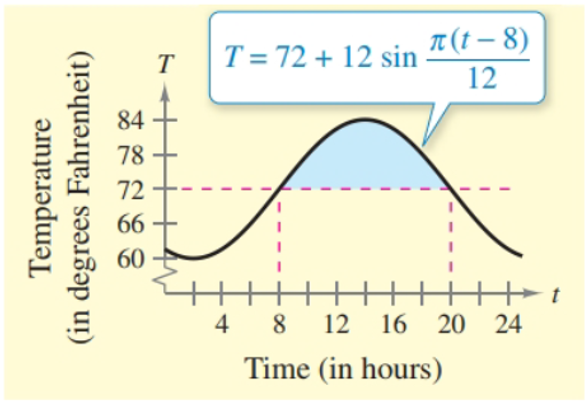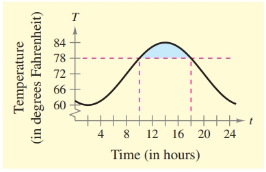Chapter 8.5, Problem 61E### Calculus: An Applied Approach (Min...

10th Edition
Ron Larson
ISBN: 9781305860919

#### Solutions

Chapter
Section### Calculus: An Applied Approach (Min...

10th Edition
Ron Larson
ISBN: 9781305860919
Textbook Problem
1 views

# Cost The temperature T (in degrees Fahrenheit) in a house is given by T = 72 + 12 sin π ( t − 8 ) 12 where t is the time (in hours), with t = 0 corresponding to midnight. The hourly cost of cooling a house is \$0.30 per degree.Find the cost C of cooling this house between 8 A.M. and 8 P.M. when the thermostat is set at 72°F (see figure) by evaluating the integral C = 0.3 ∫ 8 20 [ 72 + 12 sin π ( t − 8 ) 12 − 72 ] d t .(b) Find the saving realized by resetting the thermostat to 78°F (see figure) by evaluating the integral C = 0.3 ∫ 10 18 [ 72 + 12 sin π ( t − 8 ) 12 − 78 ] d t .(a)

To determine

To calculate: The cost of cooling in house between 8 A.M to 8 P.M, when thermostat is set at 72°F.

Explanation

Given Information:

The cost of cooling in house between 8 A.M to 8 P.M can be calculated by integrating the integral of C=0.3820[72+12sinπ(t8)1272]dt, which has provided. The provided figure,

Formula used:

Write the formula for definite integral of absinudu.

absinnudu=[cosnun]ab

Calculation:

Integrate the provided integral to find the cost of cooling in house between 8 A.M to 8 P.M.

C=0.3820[72+12sinπ(t8)1272]dt

Rewrite.

C=0.3820[12sinπ(t8)12]dt

Use the above formula integral formula,

C=0.3[12cosπ(t8)12π12]820=0

(b)

To determine

To calculate: The saving after realised that thermostat set to 78°F.

### Still sussing out bartleby?

Check out a sample textbook solution.

See a sample solution

#### The Solution to Your Study Problems

Bartleby provides explanations to thousands of textbook problems written by our experts, many with advanced degrees!

Get Started

#### Find more solutions based on key concepts